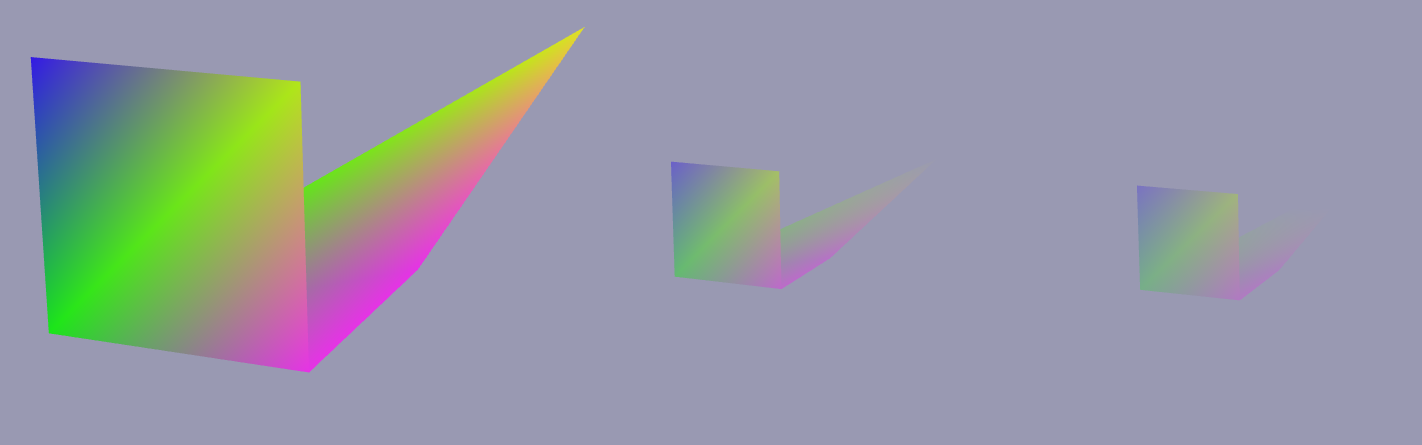Context

The provided code snippet demonstrates the basic idea behind the fog imitation. The chosen fog model is a linear function which means the fog factor increases linearly with the distance from the current camera view. The fog effect is added through a fragment shader. The GLSL shaders are provided together with the OpenSceneGraph basic code that performs a simple polygon drawing. The shaders can be used with any other OpenGL-based code provided the necessary uniforms are passed to the shaders.

Simple scene

For the scene, we will add two polygons located in two different 3D planes. We will make them adjacent one to another so that to test occlusion colors:

We also provide different colors for each of the vertices and make sure the color biding is set to BIND_PER_VERTEX:

Necessary uniforms

Just like with one of the previous shaders for drawing lines in 3D, we will need ModelViewProjectionMatrix in order to obtain gl_Position. Using OpenSceneGraph, we frame the matrix as a callback which is updated whenever current camera position changes:

Another variable that we will need is the camera’s position in 3D space at any time. Once again, we use OpenSceneGraph’s callback system to extract camera’s eye and pass it as a uniform:

The both uniforms are added to a state set (osg::StateSet) variable of a root scene:

For our purpose, we will only need to use vertex and fragment shaders. The vertex shader sets up the correct gl_Position, and the fragment shader is where we will introduce color changes in order to imitate foggy environment.

The vertex shader is the standard for GLSL version 3.3:

As an output, we make sure to pass color and 3D coordinates of each vertex. The latter will be used when we will be calculating the distance between the camera’s eye and the vertex so that to assign fog color.

We derive the fog color as a mix between the passed color of geometry and background color. In the fragment shader, we also have to pass FogColor as a uniform:

Now we can calculate the fog color based on the fragment’s location in 3D space. First, we calculate the Euclidean distance $$E()$$ between the camera eye $$C_e$$ and a 3D vertex $$V_i$$:

$d = E(C_e, V_i)$

We use the distance and plug it into our linear function for a fog to get $$\alpha$$ coefficient. The $$\alpha$$ coefficient will then be used in GLSL’s mix(x, y, alpha) function in a manner $$x(1-\alpha) + y\alpha$$. We derive $$\alpha$$ by:

$\alpha = 1 - \frac{F_{max} - d}{F_{max} - F_{min}}$

where $$F_{min}$$ and $$F_{max}$$ are the minimum and muximum distances within which the fog gradient exists. I.e., beyong the minimum distance the geometry or its part will be totally visible, while beyong the maximum distance the geometry will not be visible anymore.

For simplicity, we set up the fog thresholds inside the fragment shader; but they can also be passed as uniforms. The snippet for the fragment shader is thus:

Screenshots

Here are some screenshots of the result fog imitation:Note: the same scene is displayed but at different distances from the camera point (zoom-out operator performed).

Code snippet

Check a bit more complex example - shader-3dcurve. The fogging effect is incorporated into the fragment shader of curve shader.

Tags:

Categories:

Updated: